# Problem 4 (20 points) Consider the flow net shown below: The sides of the region are...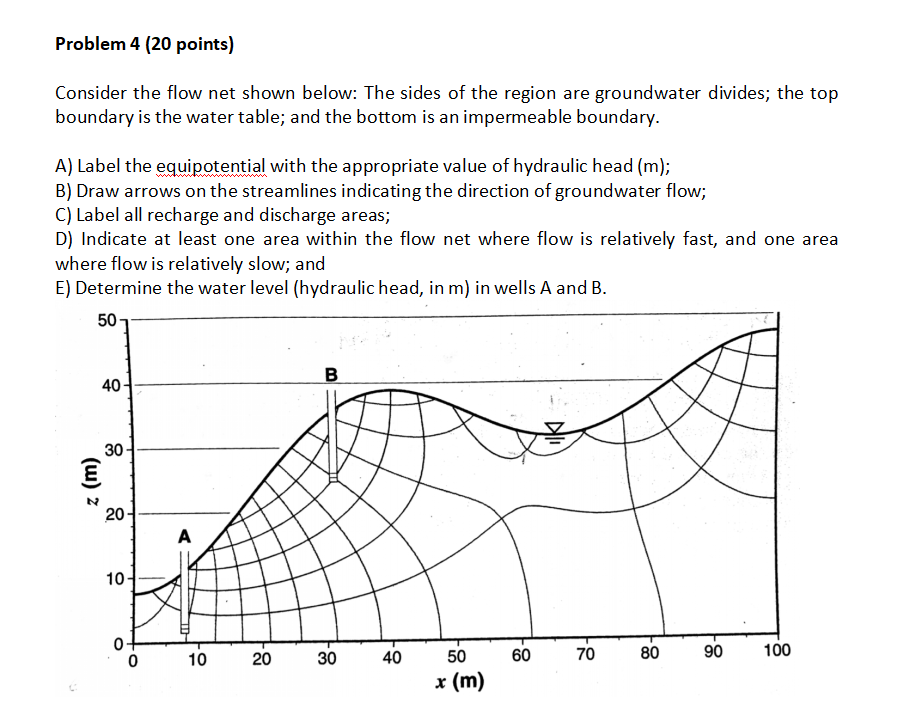Problem 4 (20 points) Consider the flow net shown below: The sides of the region are groundwater divides; the top boundary is the water table; and the bottom is an impermeable boundary. A) Label the equipotential with the appropriate value of hydraulic head (m); B) Draw arrows on the streamlines indicating the direction of groundwater flow; C) Label all recharge and discharge areas; D) Indicate at least one area within the flow net where flow is relatively fast, and one area where flow is relatively slow; and E) Determine the water level (hydraulic head, in m) in wells A and B. 50 B 40 30 { 20 A 10 10 0 20 30 40 70 100 60 80 90 50 x (m)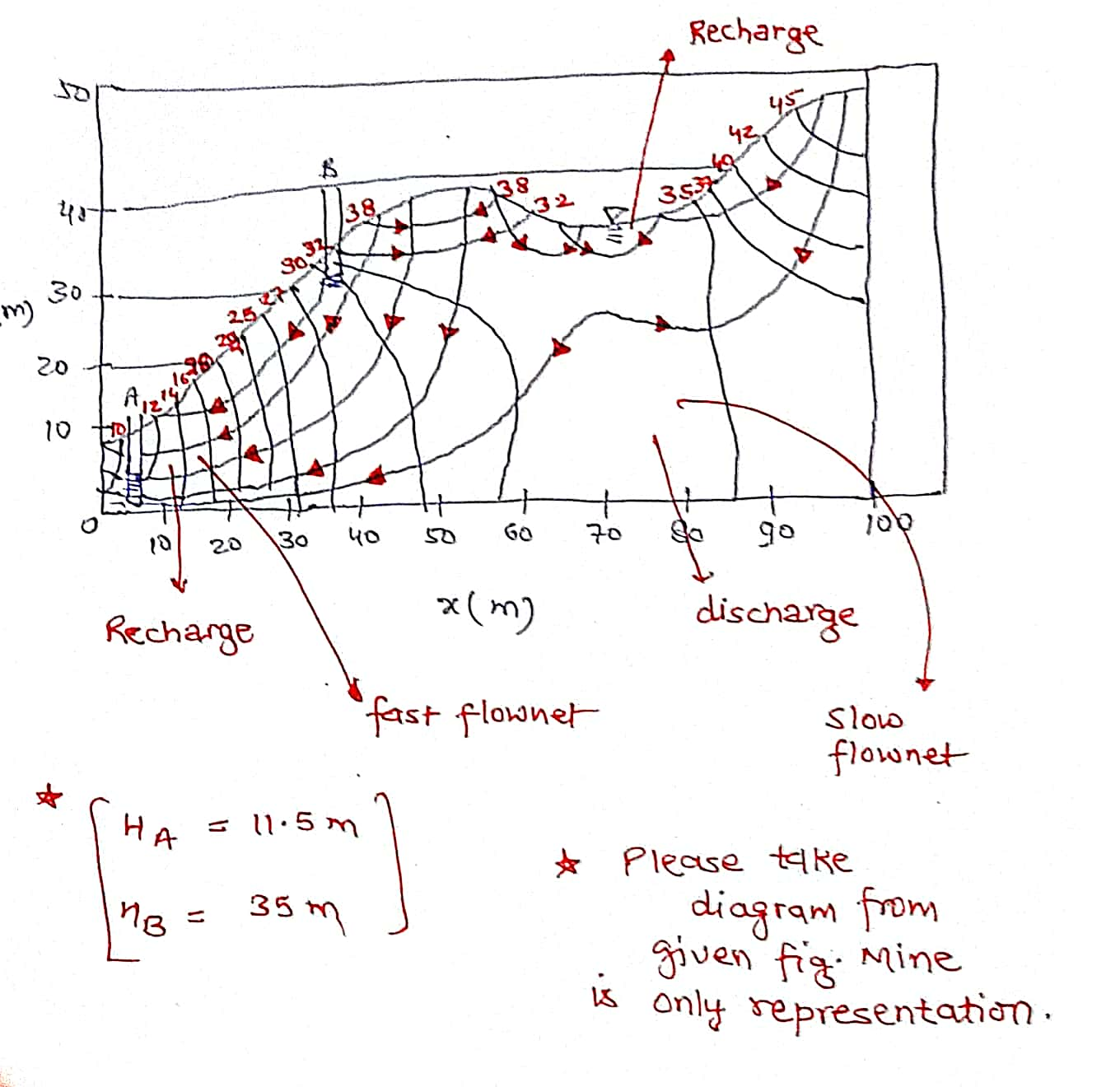#### Earn Coin

Coins can be redeemed for fabulous gifts.

Similar Homework Help Questions
• ### Consider the flow net shown below: The sides of the region are groundwater divides; the top...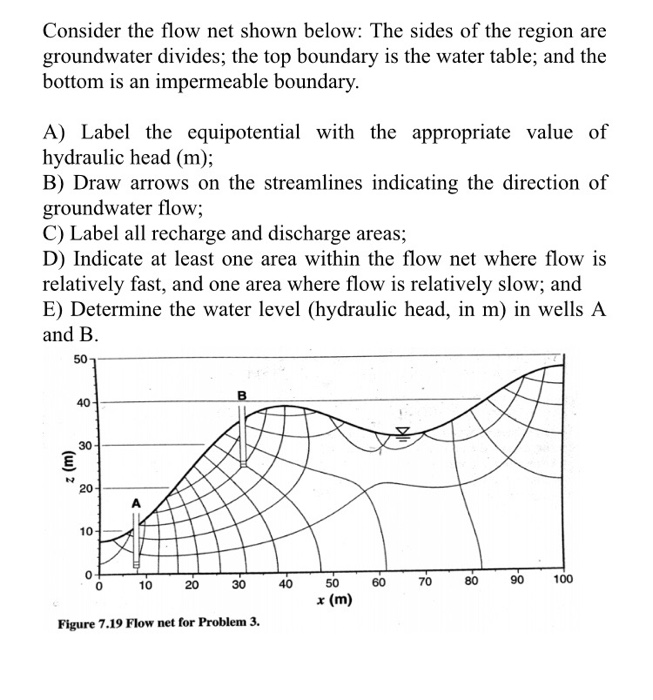Consider the flow net shown below: The sides of the region are groundwater divides; the top boundary is the water table; and the bottom is an impermeable boundary. A) Label the equipotential with the appropriate value of hydraulic head (m); B) Draw arrows on the streamlines indicating the direction of groundwater flow; C) Label all recharge and discharge areas; D) Indicate at least one area within the flow net where flow is relatively fast, and one area where flow is...

• ### For Following Multiple Choice All Correct Answers Which Applied To Each Question. 9. A flow net:...

For Following Multiple Choice All Correct Answers Which Applied To Each Question. 9. A flow net: is a diagram of equipotentials and streamlines. depicts steady groundwater flow in two dimensions. may be used to quantify groundwater flow. 10. When the hydraulic conductivity varies from point to point within an aquifer, the aquifer is said to be: homogeneous heterogeneous isotropic anisotropic 11. The Laplace equation: is a mathematical statement of the law of conservation of mass combined with Darcy’s law. may...

• ### 2. Parallel drainage canals are installed in a rectangular unconfined aquifer. They fully penetrate the aquifer and are 50 m apart. The elevation difference between the land surface and the bottom of...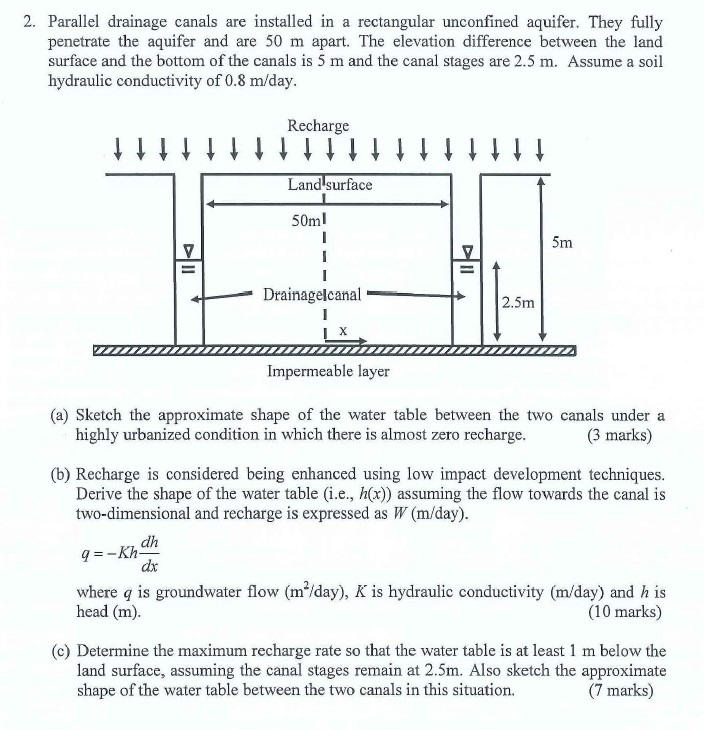2. Parallel drainage canals are installed in a rectangular unconfined aquifer. They fully penetrate the aquifer and are 50 m apart. The elevation difference between the land surface and the bottom of the canals is 5 m and the canal stages are 2.5 m. Assume a soil hydraulic conductivity of 0.8 m/day Recharge Land surface 50ml Drainagelcanal 2.5m Impermeable layer (a) Sketch the approximate shape of the water table between the two canals under a highly urbanized condition in which...

• ### Value for transmissivity is 185，location is B，flow rate is 20 Question 1: No-flow boundary conditions are implemented by: Question 2: Flow Calculation with no abstraction or rech...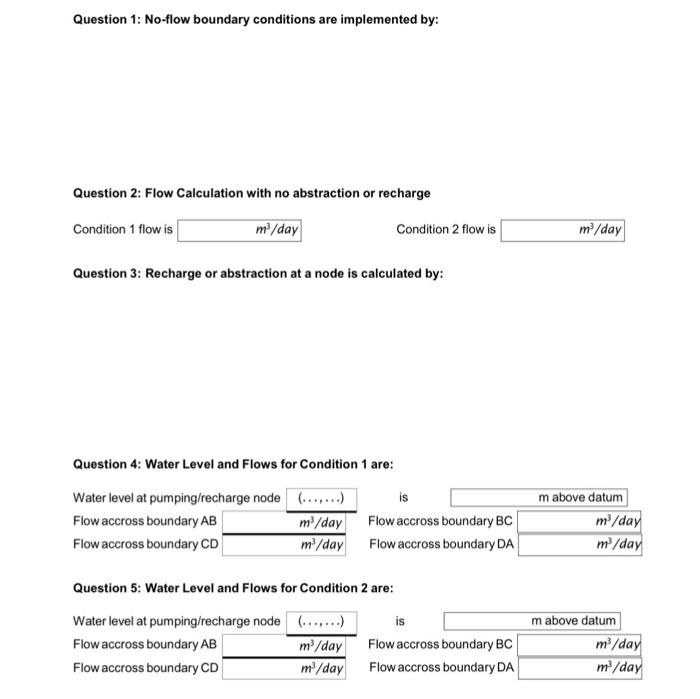Value for transmissivity is 185，location is B，flow rate is 20 Question 1: No-flow boundary conditions are implemented by: Question 2: Flow Calculation with no abstraction or recharge m2/day m/day Condition 1 flow is Condition 2 flow is Question 3: Recharge or abstraction at a node is calculated by: Question 4: Water Level and Flows for Condition 1 are: Water level at pumping/recharge node Flow accross boundary AB Flow accross boundary CD ..,..) is m3/dayFlow accross boundary BC m/dayFlow accross boundary...

• ### Q2) A canal of trapezoidal section has bed width of 8 m and bed slope of...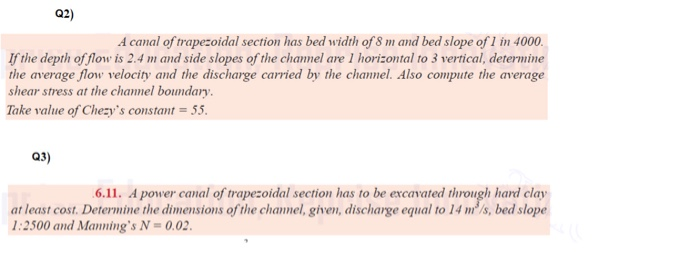Q2) A canal of trapezoidal section has bed width of 8 m and bed slope of 1 in 4000. If the depth of flow is 2.4 m and side slopes of the chamel are 1 horizontal to 3 vertical, determine the average flow velocity and the discharge carried by the channel. Also compute the average shear stress at the channel boundary. Take value of Chezy's constant = 55. Q3) 6.11. A power canal of trapezoidal section has to be excavated...

• ### *need the uplift force Problem 5 Consider the flow net shown below. The sandy soil has...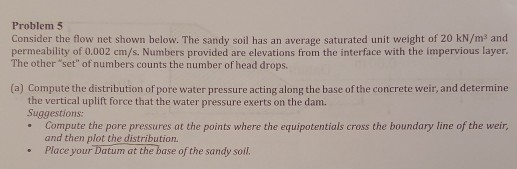*need the uplift force Problem 5 Consider the flow net shown below. The sandy soil has an average saturated unit weight of 20 kN/m and permeability of 0.002 cm/s. Numbers provided are elevations from the interface with the impervious layer. The other "set" of numbers counts the number of head drops. (a) Compute the distribution of pore water pressure acting along the base of the concrete weir, and determine the vertical uplift force that the water pressure exerts on the...

• ### 3. The flow net shown below illustrates flow conditions beneath a concrete dam featuring an upstream...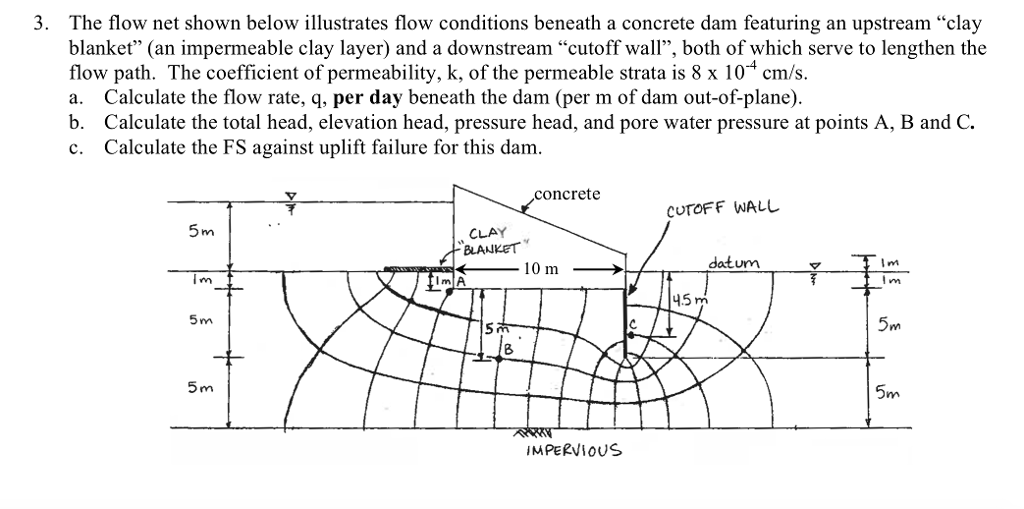3. The flow net shown below illustrates flow conditions beneath a concrete dam featuring an upstream "clay blanket" (an impermeable clay layer) and a downstream "cutoff wall", both of which serve to lengthen the flow path. The coefficient of permeability, k, of the permeable strata is 8 x 10 cm/s. a. Calculate the flow rate, q, per day beneath the dam (per m of dam out-of-plane). b. Calculate the total head, elevation head, pressure head, and pore water pressure at...

• ### A small gravity dam is founded on clayey grav els, overlying relatively impevious bedrock, as shown...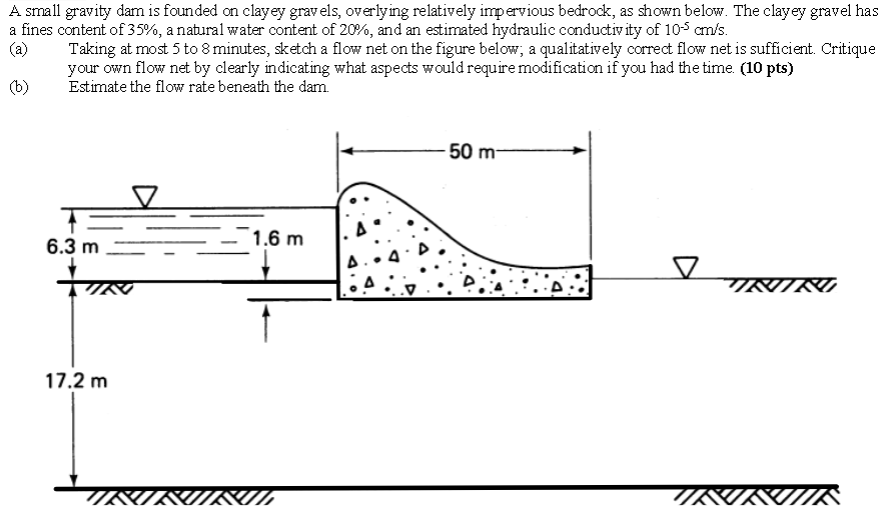A small gravity dam is founded on clayey grav els, overlying relatively impevious bedrock, as shown below. The clayey gravel has a fines content of 35%, a natural water content of 20%, and an estimated hydraulic conductivity of 10-5 ans a Taking at most 5 to 8 minutes, sketch a flow net on the figure below, a qualitatively correct flow net is sufficient. Critique your own flow net by clearly indicating what aspects would require modification if you had the...

• ### Please show all steps and work. Problem 4: (20 points) th For the hydraulic structure shown,...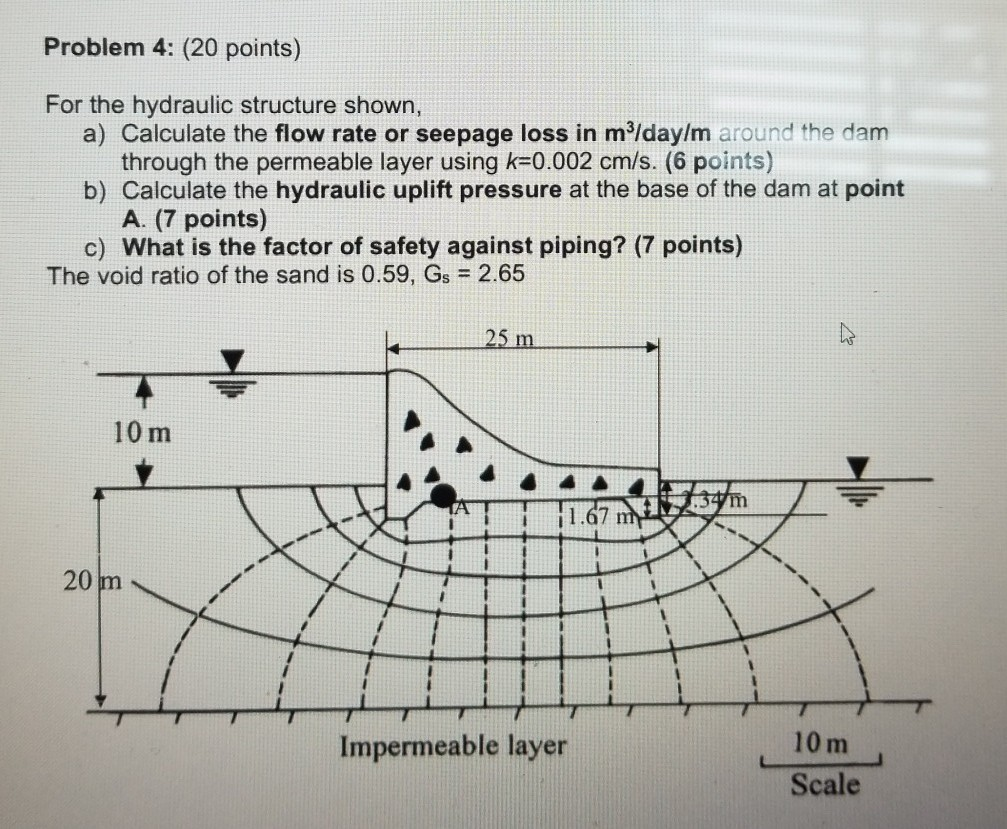Please show all steps and work. Problem 4: (20 points) th For the hydraulic structure shown, a) Calculate the flow rate or seepage loss in m3/day/m around the dam through the permeable layer using k=0.002 cm/s. (6 points) b) Calculate the hydraulic uplift pressure at the base of the dam at point A. (7 points) c) What is the factor of safety against piping? (7 points) The void ratio of the sand is 0.59, Gs = 2.65 sm 10 m...

• ### 5. A river with 20 ppm of a non-conservative (reactive) substance and an upstream flow of...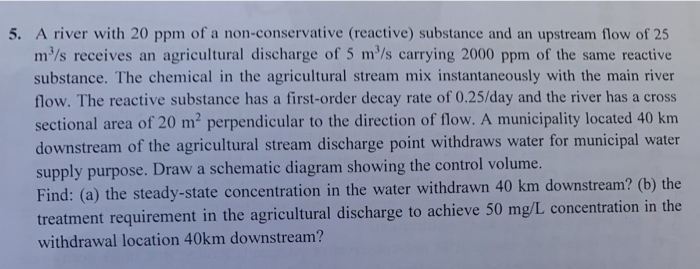5. A river with 20 ppm of a non-conservative (reactive) substance and an upstream flow of 25 m/s receives an agricultural discharge of 5 m/s carrying 2000 ppm of the same reactive substance. The chemical in the agricultural stream mix instantaneously with the main river flow. The reactive substance has a first-order decay rate of 0.25/day and the river has a cross sectional area of 20 mperpendicular to the direction of flow. A municipality located 40 km downstream of the...